Dependent (Controlled) Sources

Our previous discussion of ideal voltage and current sources exclusively considered independent sources, which had a fixed specified voltage or current regardless of whatever else was going on in a circuit.

In this section, we'll now consider dependent sources, which have a voltage or current value that is controlled by some voltage or current value elsewhere in the circuit.

In particular, we'll consider linear dependent sources, which have a value proportional to their controlling value, related only by some scaling constant.

These dependent sources are important to understand because they're how we model and analyze more complicated components, like transistors. Many transistor situations can be modeled as though a small controlling current or voltage signal at one terminal can control a much larger current or voltage at another terminal.

Voltage and current are the two primary state variables in an electronic system. Just as there are two kinds of independent sources (voltage and current), there are four basic types of dependent sources: their output can be either voltage or current, AND their input can be either voltage or current.

Each dependent source has two output terminals, just like an independent source would.

Each dependent source also has two input terminals, thought these are not always explicitly shown. For voltage controlled sources these act like an open-circuit voltage measurement in parallel with the controlling voltage. For current controlled sources these act like a short-circuit current measurement in series with the controlling current.

Voltage Controlled Voltage Source (VCVS)

A VCVS is a voltage source where the voltage is controlled by a voltage elsewhere in a circuit.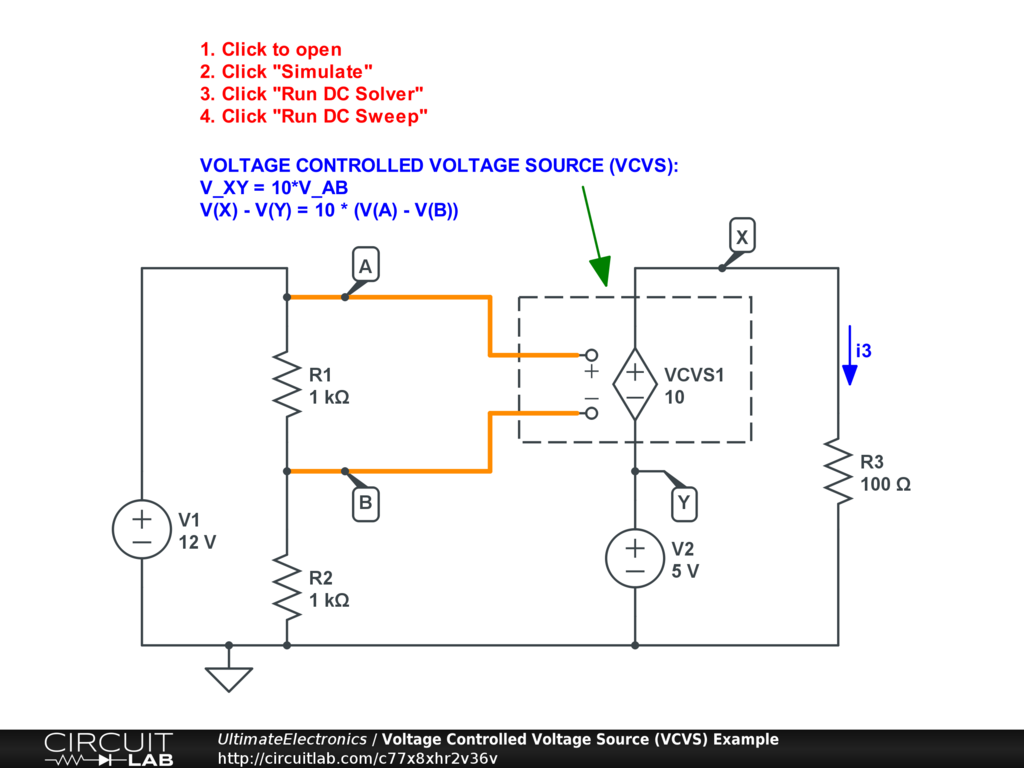Interactive Exercise Click the circuit, click "Simulate," then "Run DC Sweep" simulation.

The VCVS1 element is set with a gain of 10, so it creates an equation:

\begin{align} V_{X Y} & = 10 V_{A B} \\ \text{V(X)} - \text{V(Y)} & = 10 \cdot \big( \text{V(A)} - \text{V(B)} \big) \end{align}

As discussed in Systems of Equations and Solving Circuit Systems, we commonly write linear systems with all multiplicative terms on the left side, and just a single constant on the right hand side:

\begin{align} \text{V(X)} - \text{V(Y)} - 10 \cdot \big( \text{V(A)} - \text{V(B)} \big) & = 0 \\ \text{V(X)} - \text{V(Y)} - 10 \text{V(A)} + 10 \text{V(B)} & = 0 \end{align}

All of our linear controlled sources will have zero right-hand-side term.

This example circuit is solvable by inspection because there is no feedback between the controlling side and the controlled side of the circuit.

Since no current flows into the controlling measurement terminals of VCVS1, then V1, R1, and R2 form a simple resistive voltage divider, with $V_A=12$ and $V_B=6$, so $V_{A B}=6$.

Because of the VCVS relationship, the source will look like a voltage of $10 V_{A B}$, which is just 60 in this case. From there, we can solve the right hand side directly: $V_Y=5$ and $V_{X Y}=60$, so $V_X = 65$ and a current of $i_3 = 0.65 \ \text{A}$ flows through resistor R3.

In other circuits there will be an interconnection that makes the system harder to solve, but when there is no feedback, systems with controlled sources can be solved from input to output.

In practice, a VCVS is often used in modeling operational amplifiers (op-amps), and can also be used in modeling a voltage signal chain more generally.

Voltage Controlled Current Source (VCCS)

A VCCS is a current source where the current is controlled by a voltage elsewhere in a circuit.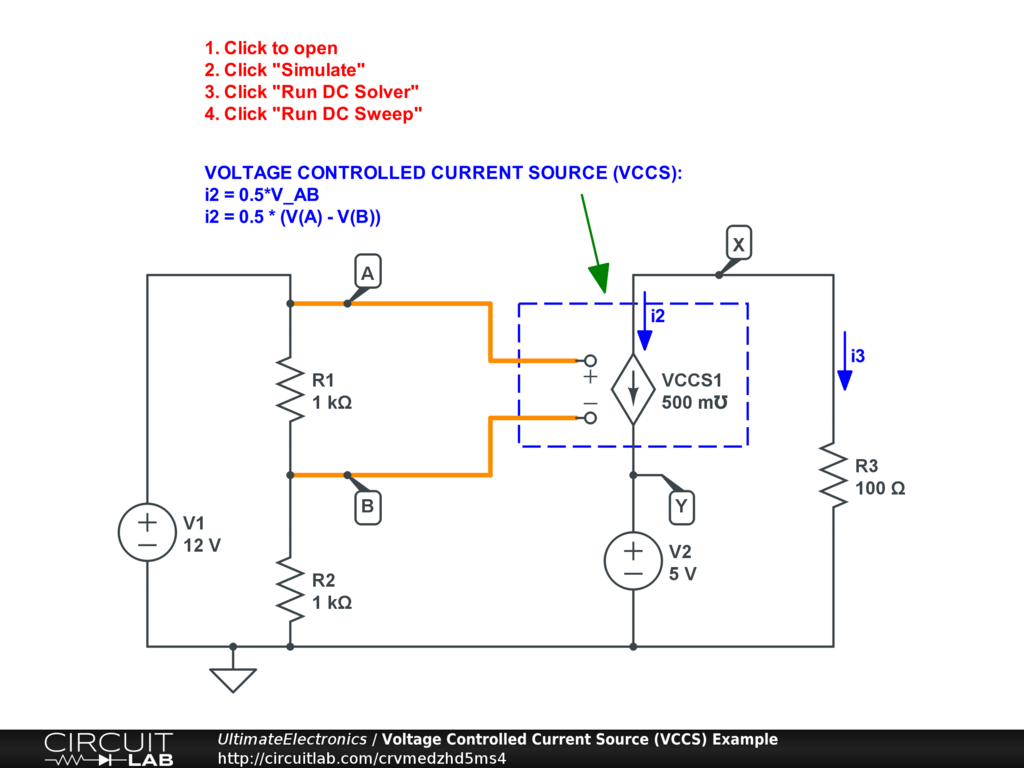Interactive Exercise Click to simulate the circuit above.

We've replaced our VCVS above with a VCCS with gain 0.5, meaning that for every $1 \ \text{V}$ of voltage difference at the input terminals, the current source will have a current of $0.5 \ \text{A}$ in the direction of the arrow as drawn. This is the branch current $i_2$ as labeled. (For a review see Labeling Voltages, Currents, and Nodes.)

\begin{align} i_2 & = 0.5 V_{A B} \\ i_2 & = 0.5 \cdot \big( \text{V(A)} - \text{V(B)} \big) \end{align}

Or collecting terms on the left-hand side:

$$i_2 - 0.5 \text{V(A)} + 0.5 \text{V(B)} = 0$$

Again, this circuit has no feedback and is simple enough to solve by inspection. $V_{A B} = 6$ as before, so $i_2 = 3$. To map to our resistor current as labeled $i_3$ we simply have to observe that $i_3 = -i_2$, so $i_3 = -3 \ \text{A}$.

If we're interested in node voltages as well, we'd simply use Ohm's Law on R3 and see that $V_X = -300$, while it remains true that $V_Y = 5$ due to voltage source V2.

In practice, a VCCS is often used in modeling MOSFETs or other voltage-effect-based transistors.

Current Controlled Voltage Source (CCVS)

A CCVS is a voltage source where the voltage is controlled by a current elsewhere in a circuit. Here, we've specified a gain of 100, and a controlling current of R1.nA, meaning the current into terminal nA of resistor R1: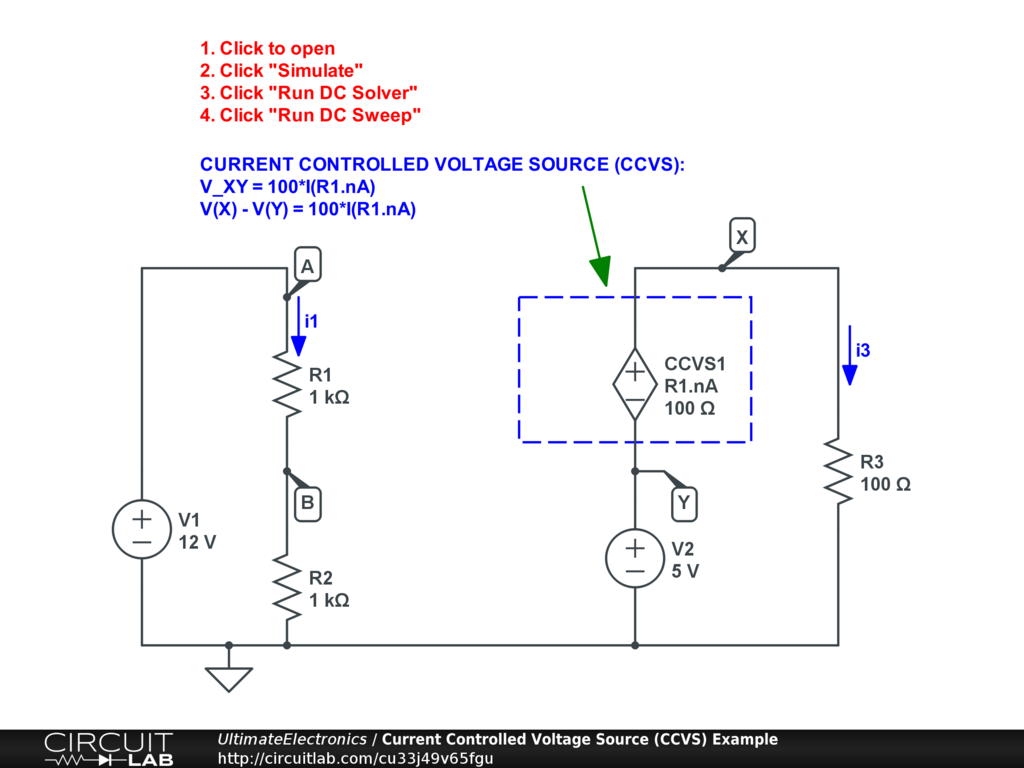Interactive Exercise Click the circuit, click "Simulate", and "Run DC Sweep" simulation.

\begin{align} V_{X Y} & = 100 \cdot \text{I(R1.nA)} \\ \text{V(X)} - \text{V(Y)} & = 100 i_1 \\ \text{V(X)} - \text{V(Y)} - 100 i_1 & = 0 \end{align}

Again, with no feedback, the total current on the left-hand side is $i_1 = \frac {12} {1000+1000} = 0.006$, so the source will be $V_{X Y} = 0.6$. This makes the total voltage $V_X = 5.6$, so $i_3 = 0.056$.

In CircuitLab you must specify the terminal current of interest. For a review of how to specify branch currents and terminal currents, please see Labeling Voltages, Currents, and Nodes.

Current Controlled Current Source (CCCS)

A CCVS is a current source where the current is controlled by some other current elsewhere in a circuit: Here we've defined a gain of 0.5 and again are using the same controlling terminal current of R1.nA.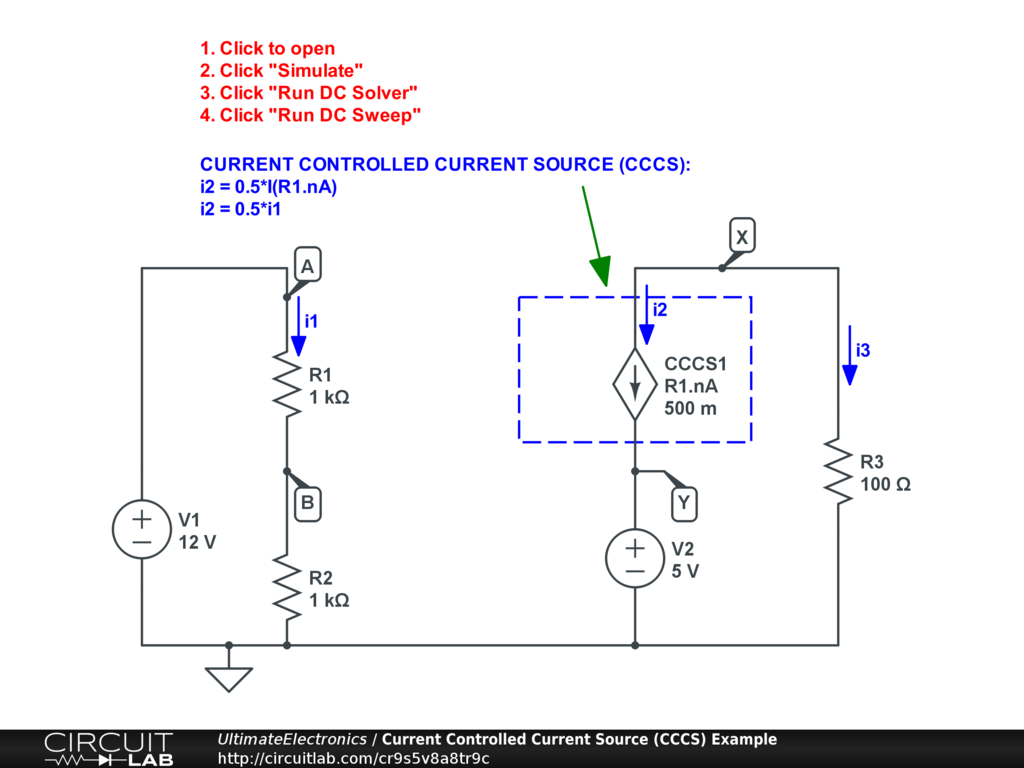Interactive Exercise Click the circuit, click "Simulate", and "Run DC Sweep" simulation.

\begin{align} i_2 & = 0.5 i_1 \\ i_2 - 0.5 i_1 = 0 \end{align}

With still no feedback, the total controlling current is unchanged $i_1 = 0.006$, so the source will be $i_2 = 0.003$. We can map to $i_3 = -i_2 = -0.003$. From there, the voltage $V_X = -0.3$.

In practice, a CCCS is often used in modeling Bipolar Junction Transistors (BJTs) or other current-effect-based transistors.

Behavioral Sources for Controlled Sources

While we can use special symbols to draw controlled sources, we can also use the special ability of all CircuitLab voltage and current sources to reference quantities elsewhere in the circuit. For example, instead of using the special VCVS symbol and connecting it as shown above, we can instead use a normal voltage source and write the expression "10*(V(A) - V(B))" as the value. This will have the same effect: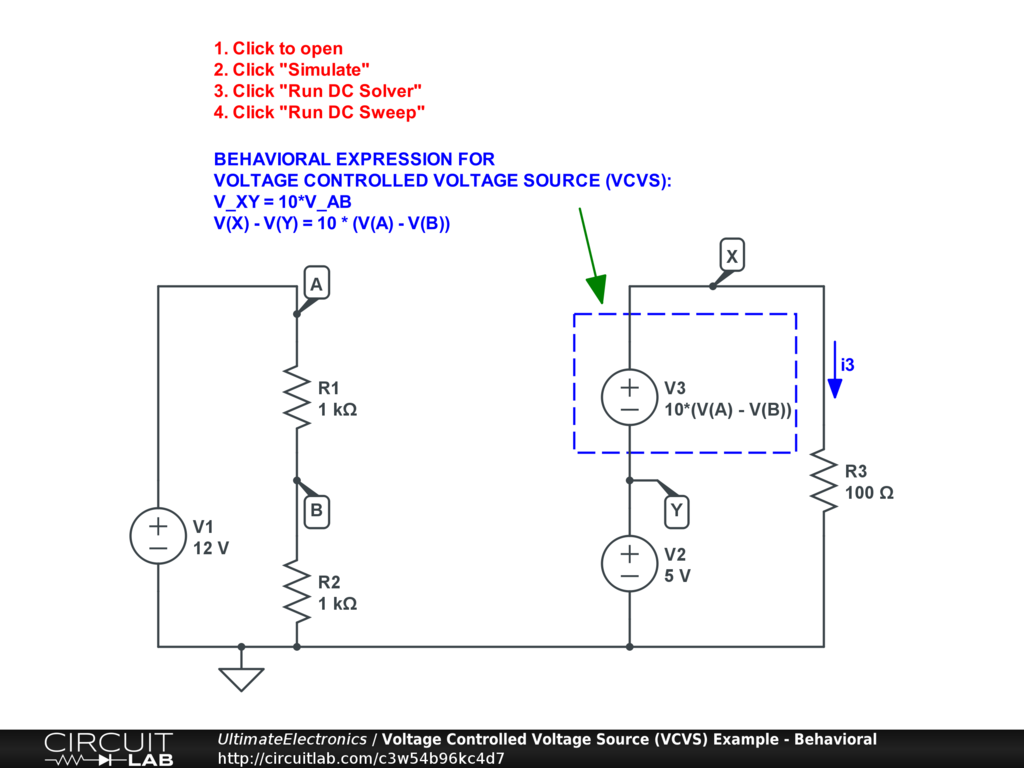Interactive Exercise You can click this and run the simulation and find that it performs identically to the VCVS circuit drawn above.

Similarly, to reference a current, we can just use I(R1.nA) notation, for example by setting a current source's value to "0.5*I(R1.nA)":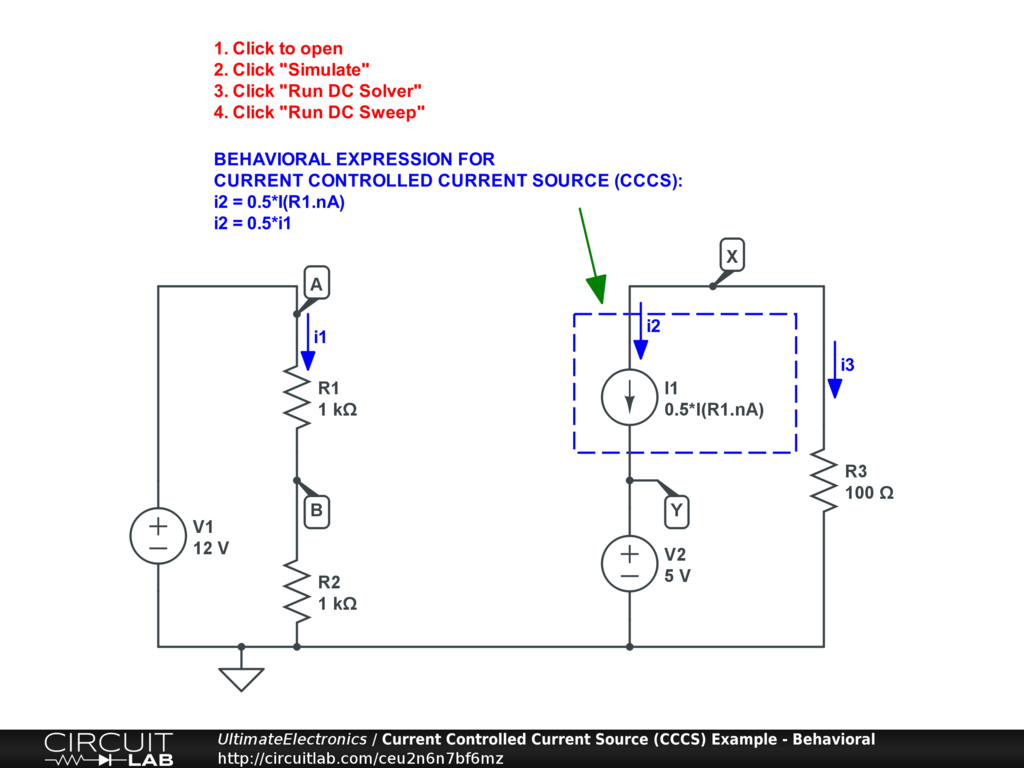Interactive Exercise Click and simulate to see that this performs identically to the CCCS circuit drawn above.

This behavioral expression approach allows for the construction of far more complicated component behaviors as we'll see in later sections.

Self-Connected Controlled Sources Are Resistors

This circuit demonstrates a VCCS connected to itself, where its own voltage determines its current. As confusing as that sounds, that's exactly what a resistor does: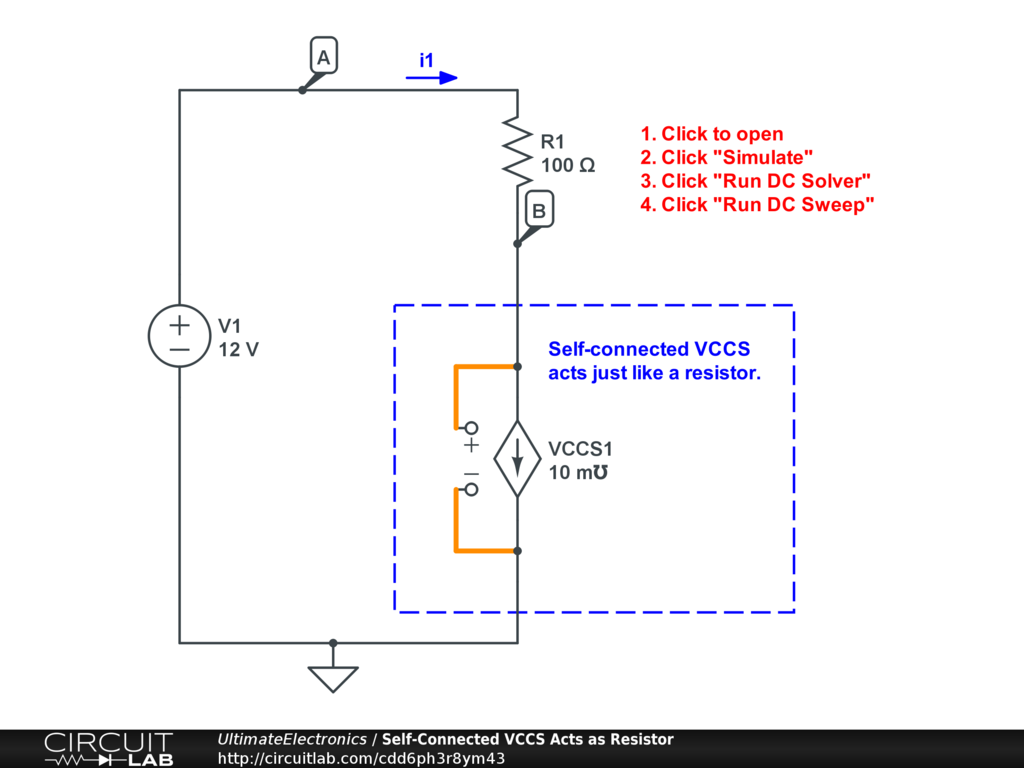Interactive Exercise Click the circuit and run the DC and DC Sweep simulations to see that VCCS1 behaves just like a $100 \ \Omega$ resistor.

The component VCCS1 obeys the following equation:

$$i_1 = 0.01 \cdot V_B$$

This is identical to a resistor, $V_B = 100 \cdot i_1$.

Similarly, the same situation can be constructed using a behavioral current source where the special function $\text{V()}$ which refers to a source's own voltage difference. Here, we replace VCCS1 with a current source I1 with a defined current $0.01 \cdot \text{V()}$.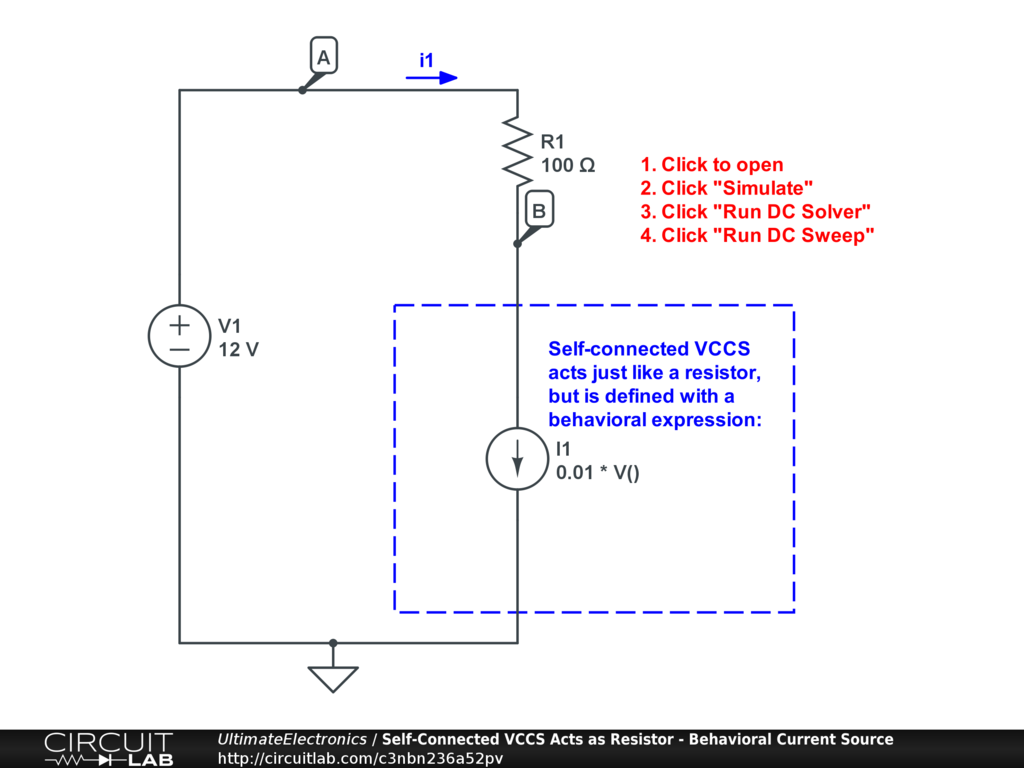Interactive Exercise Click and run this circuit to see that I1 also acts just like a resistor.

We can do the same with a self-connected CCVS as well: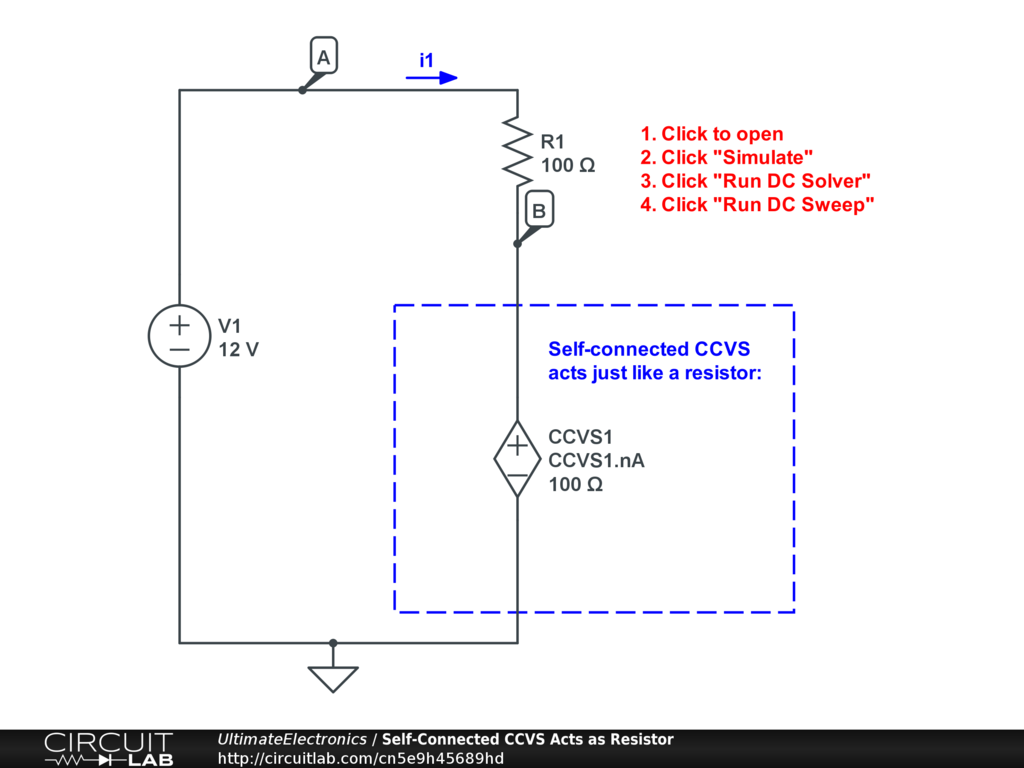Interactive Exercise Click to simulate the circuit above.

Here, the component CCVS1 refers to its own current to determine its voltage.

And we can do the same using a behavioral voltage source, using the special function $I()$ to refer to the element's own current.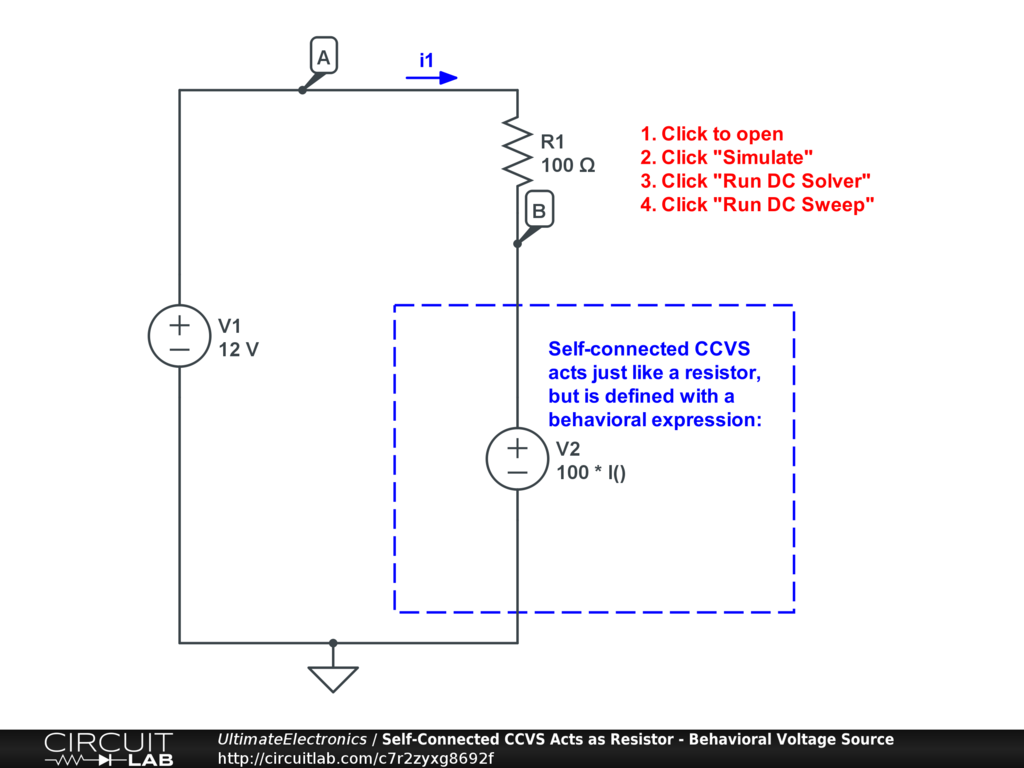Interactive Exercise Click to simulate the circuit above.

Here, we've just shown four new ways to implement a resistor! The big idea here is that from a circuit analysis perspective, resistors are just a special case where the voltage and current relationship happens to be on a single circuit element. In general, controlled sources allow for relationships to exist between various currents and voltages without requiring that they be related on the same element, and these can be expressed either with the four VCVS/VCCS/CCVS/CCCS source elements, or with current or voltage sources defined with behavioral expressions.

What's Next

In the next section, Dependent Source Feedback, we'll look at what happens when the input and output of a dependent source interact with each other.